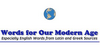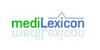noun a capacitance unit equal to one billion farads
Found on https://www.encyclo.co.uk/local/20974abfarad (aF) An abfarad is an electromagnetic unit of capacitance equal to 109 farads (1,000,000,000 F or 1 GF). This very large unit is usually limited to applications in medical terminology. A farad is a basic unit of capacitance in the meter-kilogram-second system; equivalent to the capacitance of a capacitor in which a charge of 1 cou...
Found on http://www.wordinfo.info/words/index/info/view_unit/4266/Electromagnetic unit of capacity equal to 109 farads. ... (05 Mar 2000) ...
Found on http://www.encyclo.co.uk/local/20973The CGS unit of capacitance equal to 1.0x109Farad.ttle='Abfarad';xiunt='farad';yiunt='abfarad';mconv=1e9;cconv=0.0;Type: Term Pronunciation: ab-far′ad Definitions: 1. Electromagnetic unit of capacity equal to 109 farads.[n] - a capacitance unit equal to one billion farads